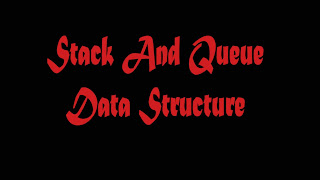# Stack And Queue Data Structures## Stacks and Queues

These types of data structures are special cases of lists. Stack also called LIFO (Last In First Out) list. In this structure items can be added or removed from only one end. Stacks are generally represented either in array or in singly linked list and in both cases insertion/deletion time is O(1), but search time is O(n).

### Operations on stacks

boolean isEmpty ();
Return true if and only if this stack is empty. Complexity is O(1).
int getLast ();
Return the element at the top of this stack. Complexity is O(1).
void clear ();
Make this stack empty. Complexity is O(1).
void push (int elem);
Add elem as the top element of this stack. Complexity is O(1).
int   pop ();
Remove and return the element at the top of this stack. Complexity is O(1).

The queues are also like stacks but they implement FIFO(First In First Out) policy. One end is for insertion and other is for deletion. They are represented mostly circularly in array for O(1) insertion/deletion time. Circular singly linked representation takes O(1) insertion time and O(1) deletion time. Again Representing queues in doubly linked list have O(1) insertion and deletion time.

### Operations on queues

boolean isEmpty ();
Return true if and only if this queue is empty. Complexity is O(1).
int size ();
Return this queue’s length. Complexity is O(n).
int getFirst ();
Return the element at the front of this queue. Complexity is O(1).
void clear ();
Make this queue empty. Complexity is O(1).
void insert (int elem);
Add elem as the rear element of this queue. Complexity is O(1).
int delete ();
Remove and return the front element of this queue. Complexity is              O(1).